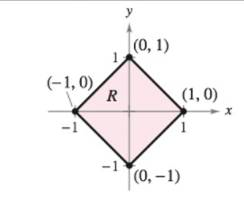Chapter 14.8, Problem 17E

Chapter
Section
Textbook Problem

Evaluating a Double Integral Using a Change of Variables In Exercises 17-22, use the indicated change of variables to evaluate the double integral. ∫ R ∫ 4 ( x 2 + y 2 )   d A x = 1 2 ( u + v ) y = 1 2 ( u − v )To determine

To calculate: The value of double integral R4(x2+y2)dA using the indicated change of variables.

Explanation

Given: The provided double integral R4(x2+y2)dA

The equations, x=12(u+v) and y=12(uv).

The following graph:

Formula used:

With the help of Jacobian’s formula δ(x,y)δ(u,v)=|δxδuδxδvδyδuδyδv|

And change of variables for double integrals is given as

Rf(x,y)dxdy=Sf(g(u,v),h(u,v))|δ(x,y)δ(u,v)|dudv

Calculation: Find the value of the Jacobian as shown below.

δ(x,y)δ(u,v)=|δxδuδxδvδyδuδyδv|δ(x,y)δ(u,v)=|12121212|δ(x,y)δ(u,v)=12

Put different values of x and y coordinates from the given graph and replace them in the given 2 equations.

Substitute (x,y)=(0,1) to get,

0=12(u+v) And 1=12(uv)

In the same way, substitute,

(x,y)=(1,0)(x,y)=(0,1)(x,y)=(1,0)

Thus, different values of u and are:

(u,v)=(1,1)(u,v)=(1,1)(u,v)=(1,1)(u,v)=(1,1)

The graph obtained is,

Still sussing out bartleby?

Check out a sample textbook solution.

See a sample solution

The Solution to Your Study Problems

Bartleby provides explanations to thousands of textbook problems written by our experts, many with advanced degrees!

Get Started

In Exercises 1728, use the logarithm identities to obtain the missing quantity.

Finite Mathematics and Applied Calculus (MindTap Course List)

If f(1) = 12, f is continuous, and 14f(x)dx=17, what is the value of f(4)?

Single Variable Calculus: Early Transcendentals, Volume I

Evaluate the limit. limx0tan4xx+sin2x

Single Variable Calculus: Early Transcendentals

Find each product: (x+3)2

Elementary Technical Mathematics